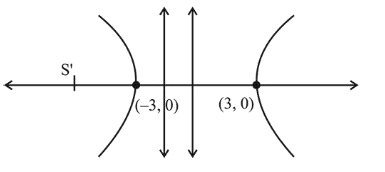# If 5 x+9=0 is the directrix of the hyperbola

Question:

If $5 x+9=0$ is the directrix of the hyperbola $16 x^{2}-9 y^{2}=144$, then its corresponding focus is :

1. (1) $(5,0)$

2. (2) $\left(-\frac{5}{3}, 0\right)$

3. (3) $\left(\frac{5}{3}, 0\right)$

4. (4) $(-5,0)$

Correct Option: , 4

Solution:

$16 x^{2}-9 y^{2}=144 \Rightarrow \frac{x^{2}}{9}-\frac{y^{2}}{16}=1$

Then focus is S' $(-a e, 0)$$x=\frac{-9}{5}$

$\mathrm{a}=3, \mathrm{~b}=4 \Rightarrow e^{2}=1+\frac{16}{9}=\frac{25}{9} \quad\left[\because e=\sqrt{1+\frac{b^{2}}{a^{2}}}\right]$

$\therefore$ the focus $S^{\prime} \equiv\left(3-\times \frac{5}{3}, 0\right) \equiv(-5,0)$